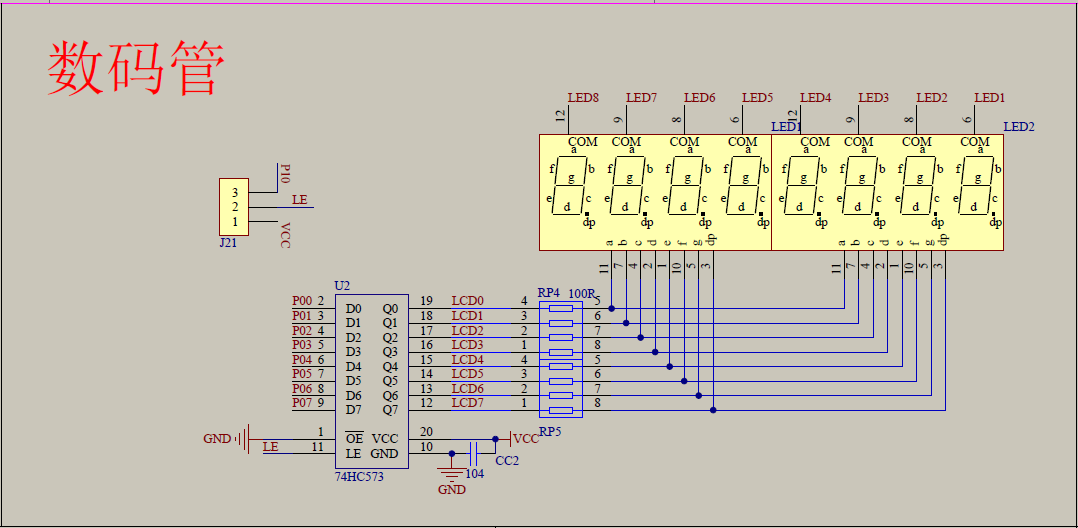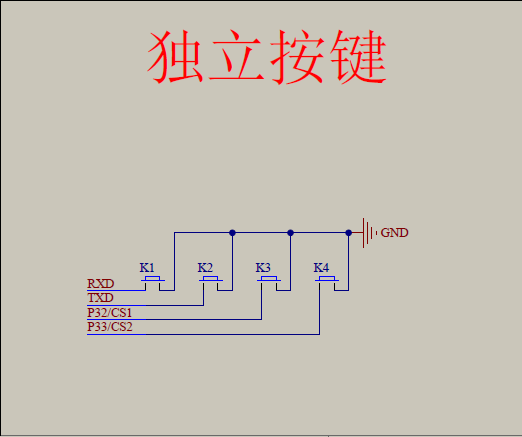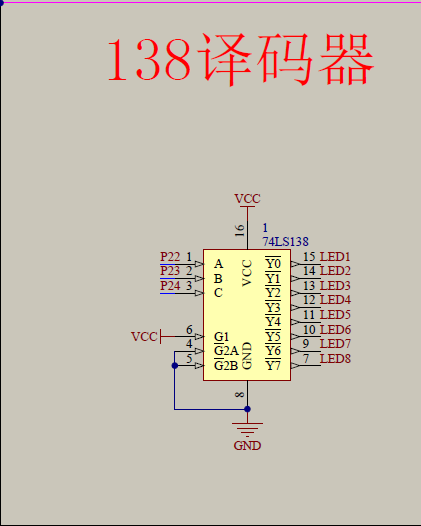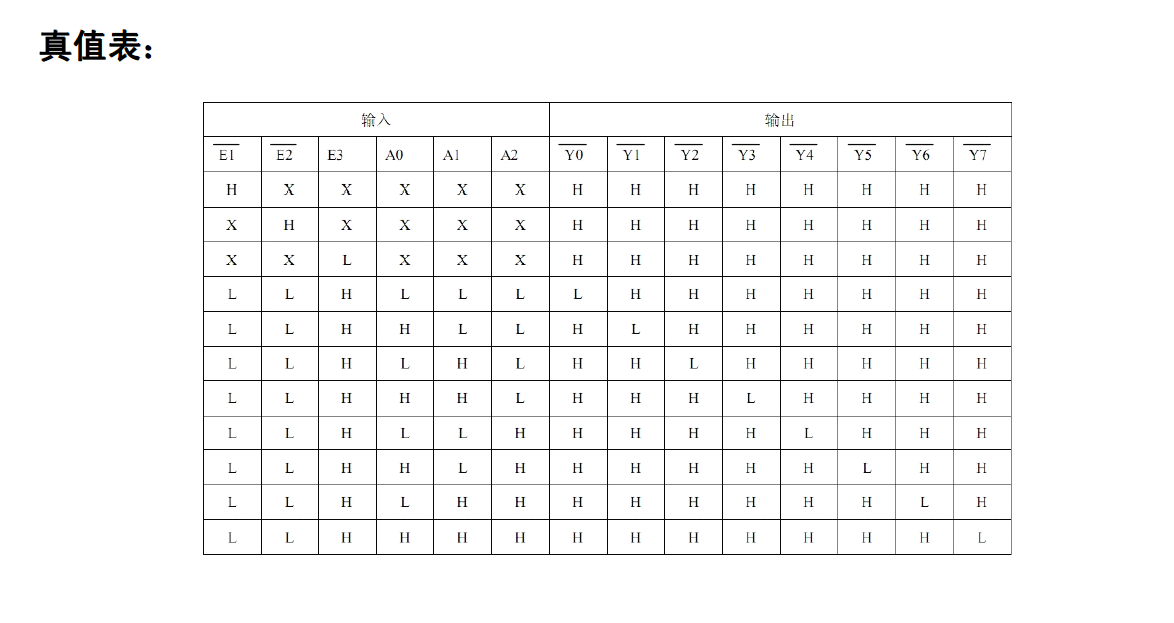2015-11-19 16:11:42 cjdsn 阅读数 1550
• ###### 51单片机综合小项目-第2季第4部分

本课程是《朱有鹏老师单片机完全学习系列课程》第2季第4个课程，也是51单片机学完之后的一个综合小项目，该项目运用了开发板上大多数外设设备，并将之结合起来实现了一个时间、温度显示以及报警功能、时间调整功能等单片机控制常见的功能，有一定代码量，需要一定调试技巧和编程能力来完成，对大家是个很好的总结和锻炼，并且能拓展项目经验。

3414 人正在学习 去看看 朱有鹏
/*
write by cjdsn
时间计算
1/22.1184=0.0000005425
1/0.5425=1843317
1843317/40=46083
//我的是阳极显示，0开1关
*/

#include<reg52.h>
unsigned char code Tab=

{0xc0,0xf9,0xa4,0xb0,0x99,0x92,0x82,0xf8,0x80,0x90,0x7f,0xff};
//数码管显示0～9的段码表
unsigned char int_time;        //记录中断次数
unsigned char second;        //储存秒
unsigned char t,h;
sbit pd=P2^0;
sbit pc=P2^1;
sbit pb=P2^2;
sbit pa=P2^3;
sbit p27=P2^7;
/******************************************************************

*****

*******************************************************************

*****/
void delay(void)
{
unsigned char i;
for(i=0;i<200;i++)
;
}
/******************************************************************

*****

*******************************************************************

*****/
void DisplaySecond(unsigned char k)
{
pb=0;        //P2.1引脚输出低电平
P0=Tab[k/10];          //显示十位
delay();
delay();
delay();
delay();
delay();
delay();
delay();
delay();
pb=1;
pa=0;      //P2.0引脚输出低电平
P0=Tab[k%10];         //显示个位
delay();
delay();
delay();
delay();
delay();
delay();
delay();
delay();
pa=1;
pd=0;
P0=Tab[t/10];  //分 十位
delay();
delay();
delay();
delay();
delay();
delay();
delay();
delay();
pd=1;
pc=0;
P0=Tab[t%10];  //分 个位
delay();
delay();
delay();
delay();
delay();
delay();
delay();
delay();
pc=1;
///////////////////////////////////
pc=0;
P0=Tab;
delay();
delay();
delay();
delay();
delay();
delay();
pc=1;
//====================================//
/*
P2=0xff;     //关闭所有数码管
P0=0xff;        //显示个位
*/
//====================================//
}

///////////////////////////////////////////////////////////////////

/////////////////

void Displayh()
{
pb=0;        //P2.1引脚输出低电平
P0=Tab[t/10];          //显示十位
delay();
delay();
delay();
delay();
delay();
delay();
delay();
delay();
pb=1;
pa=0;      //P2.0引脚输出低电平
P0=Tab[t%10];         //显示个位
delay();
delay();
delay();
delay();
delay();
delay();
delay();
delay();
pa=1;
pd=0;
P0=Tab[h/10];  //分 十位
delay();
delay();
delay();
delay();
delay();
delay();
delay();
delay();
pd=1;
pc=0;
P0=Tab[h%10];  //分 个位
delay();
delay();
delay();
delay();
delay();
delay();
delay();
delay();
pc=1;
///////////////////////////////////
pc=0;
P0=Tab;
delay();
delay();
delay();
delay();
delay();
delay();
pc=1;
}

////////////////////////////////  显示小数点
displayms(void)
{
P2=1;
p27=1;
pa=0;
pb=1;
P0=Tab;
delay();
delay();
delay();
delay();
delay();
delay();
delay();
delay();
delay();
delay();
delay();
delay();
delay();
delay();
pa=1;
}

//==========================================================//

void main(void)
{
P2=1;
p27=1;
TMOD=0x01;                 //使用定时器T0
TH0=(-46083)/256;    //将定时器计时时间设定为

46083×1.085微秒
//=50000微秒=50毫秒
TL0=(-46083)%256;
EA=1;
ET0=1;
TR0=1;
int_time=0;     //中断次数初始化
second=0;   //秒初始化
t=59;

while(1)
{       if(h<=0){
DisplaySecond(second);//调用秒的显示子程序
}
else Displayh();
}
}
//********************************************************
//函数功能：定时器T0的中断服务程序
//*******************************************************
void interserve(void ) interrupt 1 using 1
{
TR0=0;    //关闭定时器T0
int_time ++;        //每来一次中断,中断次数int_time自加1

if(int_time==40)   //够20次中断,即1秒钟进行一次检测结果采样
{
int_time=0;    //
second++;    //秒加1
if(h>0){displayms();}
if(second==60)
{  second =0; //秒等于60就返回0
t++;}
if(t==60){t=0;h++;
if(h==24)h=0;}
}
TH0=(-46083)/256;   //重新给计数器T0赋初值
TL0=(-46083)%256;
TR0=1;     //启动定时器T0
}

2019-05-18 00:18:34 jianfeng_520 阅读数 1573
• ###### 51单片机综合小项目-第2季第4部分

本课程是《朱有鹏老师单片机完全学习系列课程》第2季第4个课程，也是51单片机学完之后的一个综合小项目，该项目运用了开发板上大多数外设设备，并将之结合起来实现了一个时间、温度显示以及报警功能、时间调整功能等单片机控制常见的功能，有一定代码量，需要一定调试技巧和编程能力来完成，对大家是个很好的总结和锻炼，并且能拓展项目经验。

3414 人正在学习 去看看 朱有鹏

## 说明

74HC138译码器可接受3位二进制加权地址输入（A0, A1和A2），并当使能时，提供8个互斥的低有效输出（Y0至Y7）。74HC138特有3个使能输入端：两个低有效（E1和E2）和一个高有效（E3）。除非E1和E2置低且E3置高，否则74HC138将保持所有输出为高。74HC138正常工作的话，一次只能输出一个低电平，其他全为高电平

## 要求：

1. 按下按钮S1，秒表开始计时；
2. 按下按钮S2，秒表暂停计时（停留在计时的数）；
3. 按下按钮S3，秒表清零；
4. 计时精确到0.5s

## 电路图## 程序

/****************************************************************************************
秒表实验

*****************************************************************************************/
#include <reg52.h>
#define uint unsigned int						  //对数据类型进行声明定义
#define uchar unsigned char

sbit LS138_A = P2^2;							  //138芯片A2引脚
sbit LS138_B = P2^3;							  //138芯片A1引脚
sbit LS138_C = P2^4;							  //138芯片A0引脚

uchar DT_s = 0;								  //秒计时
uchar DT_min = 0;									  //分计时

uchar code duan[] = {0x3F,0x06,0x5B,0x4F,		  //共阴数码管无小数点 显示0~9
0x66,0x6D,0x7D,0x07,
0x7F,0x6F
};

uchar code duanpoint[] = {0xbf,0x86,0xdb,0xcf,	   //共阴数码管有小数点 显示0~9
0xe6,0xed,0xfd,0x87,
0xff,0xef,0xf7,0xfc,
0xb9,0xde,0xf9,0xf1
};

/************************************************

************************************************/
void delay1ms(uint t)
{
uint i,j;
for(i=0;i<t;i++)
{
for(j=0;j<120;j++);
}
}

/************************************************

*************************************************/
void Timer0Init()
{
TMOD = 0x01; 		//选择T0定时/计数器，工作在方式1，16位计数器
TH0 = 0xFC;			//计数初始值，计数从64536开始，计1000个数，完成一次计数，时间为1ms
TL0 = 0x18;
ET0 = 1;			//定时/计数器0中断允许位
EA = 1;				//总中断
}

/************************************************

*************************************************/
void S3()
{
DT_s = 0;			//秒归零
DT_min = 0;			//分归零
TR0 = 0;			//运行控制位清0，关闭定时器
}

/**********************************************************

**********************************************************/
void ScanKey()
{
uchar Key;		   //临时变量
Key = P3&0x07;	   //只需要三个按键，分别接的P3^1,P3^2,P3^3,故把P3高五位被屏蔽
if(Key!=0x07)	   //判断哪个键被按下
{
delay1ms(10);	 //消抖10ms
Key = P3&0x07;	 //再次赋值，避免此时按键状态改变
if(Key!=0x07)	 //确认按键被按下
{
switch(Key)
{
case 0x05:				  //S1（P3^2）被按下
TR0 = 1;	  //定时器0运行控制位为1，启动定时器0
break;
case 0x06:				  //S2（P3^1）被按下
TR0 = 0;	  //定时器0运行控制位为0，关闭定时器0
break;
case 0x03:				  //S3（P3^3）被按下
S3();
break;
}
}
while(Key!=0x07)		//松手检测
{
Key = P3&0x07;
}
}
}

/*******************************************************************

********************************************************************/
void DigDisplay(uchar s,uchar min)
{
LS138_A = 0;			  //秒个位位选
LS138_B = 0;
LS138_C = 0;
P0 = duan[s%10];		  //发送段码，显示秒个位
delay1ms(5);			  //间隔一段时间扫描
LS138_A = 1;			  //秒十位位选
LS138_B = 0;
LS138_C = 0;
P0 = duan[s/10];		  //发送段码显示秒十位
delay1ms(5);			  //间隔一段时间扫描

LS138_A = 0;			  //分的个位位选
LS138_B = 1;
LS138_C = 0;
P0 = duanpoint[min%10];		  //发送段码，显示分的个位
delay1ms(5);			  //间隔一段时间扫描
LS138_A = 1;			  //分的十位位选
LS138_B = 1;			  //间隔一段时间扫描
LS138_C = 0;
P0 = duan[min/10];		  //发送段码，显示分的十位
delay1ms(5);			  //间隔一段时间扫描
P0 = 0x00;				  //消隐
}

/**********************************************************

**********************************************************/
void main(void)
{
P0 = 0x00;		  //读端口前写1
P3 = 0xFF;		  //读端口前写1
Timer0Init();	  //定时器中断初始化函数
while(1)
{
DigDisplay(DT_s,DT_min);	   //数码管显示函数
ScanKey();							   //按键扫描函数
}
}

/**********************************************************

**********************************************************/
void Timer0() interrupt 1
{
static uint count_s;
static uint count_min;
TH0 = 0xFC;				//计数值初始化，从64536开始计数，计满时为65536，溢出时即为 1ms
TL0 = 0x18;
count_s++;				 //秒计数
count_min++;				 //分计数
if(count_s==1000)			 //计数到1s时，秒计数器开始工作
{
count_s = 0;		 //秒计数清零
DT_s++;			 //显示秒计数值自增
if(DT_s>59)		 //秒数最大为59
{
DT_s = 0;
}
}
if(count_min==60000)		  //计数到60000ms时，秒计数器开始工作
{
count_min = 0;		  //分计数清零
DT_min++;				  //显示分计数值自增
if(DT_min>59)			  //分数最大为59
{
DT_min = 0;
}
}
}


2018-01-11 09:00:12 qq_27332285 阅读数 7464
• ###### 51单片机综合小项目-第2季第4部分

本课程是《朱有鹏老师单片机完全学习系列课程》第2季第4个课程，也是51单片机学完之后的一个综合小项目，该项目运用了开发板上大多数外设设备，并将之结合起来实现了一个时间、温度显示以及报警功能、时间调整功能等单片机控制常见的功能，有一定代码量，需要一定调试技巧和编程能力来完成，对大家是个很好的总结和锻炼，并且能拓展项目经验。

3414 人正在学习 去看看 朱有鹏如果编译出错，将keil按如图参数设置：#include<1602.h>
#include<reg52.h>

#ifndef uchar
#define uchar unsigned char
#endif

#ifndef uint
#define uint unsigned int
#endif

/*按键定义*/
/*开始键，计数键，停止计数键或者清零键，上下查阅键，删除数据键*/

sbit key_start=P1^0;
sbit key_count=P1^1;
sbit key_stop=P1^2;
sbit key_up=P1^3;
sbit key_down=P1^4;

/*变量定义*/
int count=0;//计数器

uint i,j,state,k=0,countnum,m,delete;

/*设置标志位*/

char flag;
char maxflag;
char clearflag;
char turnflag; //
char upflag;  //为1时可以继续上翻
char minflag=0; //为0时可以继续下翻
char downflag;

/*定义数组 */
unsigned char str[] = "time:";
unsigned char str2[]= "num:";
unsigned char str3[]="...press start..";
unsigned char str4[]="....counting....";
unsigned char str5[]=".. press count..";
unsigned char str6[]="press up or down";
unsigned char str7[]="..overcounting..";

int number=0;
int num2=0;

void keyscan();
void init();

void main()
{

InitLcd1602();
init();
/*以下为调试程序段*/
LcdShowStr(0,0,str3);
//  LcdShowStr(0,1,str2);
//  LcdWriteCom(0x91);
//  LcdWriteData('0'+1);
//  LcdWriteCom(0x90);
//   LcdWriteData('0'+1);
/*以上为调试程序段*/

while(1)
{ //LcdShowStr(0,1,str4); //测试
keyscan();
Lcd1602_Delay1ms(50);
}

}
void init()
{
IT0=0;
EX0=0;
TMOD=0x01;
TH0=(65536-10000)/256;
TL0=(65536-10000)%256;
EA=0; //开总中断

// key_count=0;
// key_stop=0;
// key_up=0;
// key_down=0;
// key_delete=0;

flag=0; //标志位为0时代表未计时状态,为1时代表为计时状态
i=0;
maxflag=0;//定时标志位，为1时代表定时已满
clearflag=0;//清除标志位
turnflag=0;//为1时可以上下翻页显示
downflag=0;
upflag=1;
}
void keyscan()
{
if((key_start==0)&&(flag==0)) //防抖处理
{
Lcd1602_Delay1ms(10); //防抖处理
if((key_start==0)&&(flag==0))//防抖处理
{
while((!(key_start==0)&&(flag==0)));//防抖处理
InitLcd1602();
EA=1;//检测到开始按键按下后，启动定时器
ET0=1; //计时器0开启
TR0=1; //计时器打开
flag=1;//设置标志位为计时状态
LcdShowStr(0,0,str4);
LcdShowStr(0,1,str5);
}
}
if((key_count==0)&&(flag==1)&&(maxflag==0))
{
Lcd1602_Delay1ms(50);
if((key_count==0)&&(flag==1)&&(maxflag==0))
{

while(!((key_count==0)&&(flag==1)&&(maxflag==0)));

InitLcd1602();
LcdShowStr(0,0,str);
LcdShowStr(0,1,str2);
number[i]=count;
LcdWriteCom(0xC7);
LcdWriteData('0'+i+1);
LcdWriteCom(0xC6);
LcdWriteData('0');
if(i==9)
{
LcdWriteCom(0xC7);
LcdWriteData('0');
LcdWriteCom(0xC6);
LcdWriteData('0'+1);
}

num2=number[i]/10000;
num2=number[i]/1000%10;
num2=number[i]/100%10;
num2=number[i]/10%10;
num2=number[i]%10;

LcdWriteCom(0x86);
LcdWriteData('0'+num2);

LcdWriteCom(0x87);
LcdWriteData('0'+num2);

LcdWriteCom(0x88);
LcdWriteData('0'+num2);

LcdWriteCom(0x89);
LcdWriteData('.');

LcdWriteCom(0x8A);
LcdWriteData('0'+num2);

LcdWriteCom(0x8B);
LcdWriteData('0'+num2);

LcdWriteCom(0x8D);
LcdWriteData('S');

i++;
state=i;

if(i==10)
{
maxflag=1;

}
}
}

if(key_stop==0)
{
Lcd1602_Delay1ms(50);

if(key_stop==0)
{
while(!(key_stop==0));
if(clearflag==0)
{
TR0=0;  //关定时器，查阅时定时就停止
EX0=1;  //开外部中断，保证只有在上下翻阅时才能触发外部中断
count=0;//计数清零
clearflag=1;//清除标志位打开，也就是说再按一下这个按键所有制清零
turnflag=1;
minflag=0;

}
else if(clearflag==1)//此块执行清除任务
{

InitLcd1602();
number=0;
clearflag=0;
LcdShowStr(0,0,str3);
turnflag=0;
maxflag=0;
flag=0;
i=0;//将数组计数给清零
}
}
}

if(key_up==0)
{
Lcd1602_Delay1ms(50);
if(key_up==0)
{
while(!(key_up==0));
if(upflag==0)
{
InitLcd1602();
LcdShowStr(0,0,str);
LcdShowStr(0,1,str2);
minflag=0;//只要上翻过后都可以下翻
state++;
if(state==10)
{
LcdWriteCom(0xC7);
LcdWriteData('0');
LcdWriteCom(0xC6);
LcdWriteData('0'+1);
}
else
{
LcdWriteCom(0xC7);
LcdWriteData('0'+state);
LcdWriteCom(0xC6);
LcdWriteData('0');
}
m=state-1;
delete=m;

/*显示时间*/
num2=number[m]/10000;
num2=number[m]/1000%10;
num2=number[m]/100%10;
num2=number[m]/10%10;
num2=number[m]%10;

LcdWriteCom(0x86);
LcdWriteData('0'+num2);

LcdWriteCom(0x87);
LcdWriteData('0'+num2);

LcdWriteCom(0x88);
LcdWriteData('0'+num2);

LcdWriteCom(0x89);
LcdWriteData('.');

LcdWriteCom(0x8A);
LcdWriteData('0'+num2);

LcdWriteCom(0x8B);
LcdWriteData('0'+num2);

LcdWriteCom(0x8D);
LcdWriteData('S');
if(state==10)
{
upflag=1;
}
}

}

}

if(key_down==0)
{
Lcd1602_Delay1ms(50);
if(key_down==0)
{
while(!(key_down==0));
if((turnflag==1)&&(minflag==0))
{
InitLcd1602();
LcdShowStr(0,0,str);
LcdShowStr(0,1,str2);
/*显示组号*/
state--;
LcdWriteCom(0xC7);
LcdWriteData('0'+state);
LcdWriteCom(0xC6);
LcdWriteData('0');
k=state-1;
delete=k;

/*显示时间*/
num2=number[k]/10000;
num2=number[k]/1000%10;
num2=number[k]/100%10;
num2=number[k]/10%10;
num2=number[k]%10;

LcdWriteCom(0x86);
LcdWriteData('0'+num2);

LcdWriteCom(0x87);
LcdWriteData('0'+num2);

LcdWriteCom(0x88);
LcdWriteData('0'+num2);

LcdWriteCom(0x89);
LcdWriteData('.');

LcdWriteCom(0x8A);
LcdWriteData('0'+num2);

LcdWriteCom(0x8B);
LcdWriteData('0'+num2);

LcdWriteCom(0x8D);
LcdWriteData('S');

if(state==1)
{
minflag=1;

}
upflag=0;

}
}
}

}

void timer0() interrupt 1
{
TH0=(65536-10000)/256;
TL0=(65536-10000)%256;
count++;
if(count==6000)
{
count=0;
ET0=0; //计时器0关闭
TR0=0; //计时器关闭
turnflag=1;
EX0=1;
InitLcd1602();
LcdShowStr(0,0,str6);
LcdShowStr(0,1,str7);
}
}

void  counter0(void) interrupt 0  using 1
{
number[delete]=0;
LcdWriteCom(0x86);
LcdWriteData('0');

LcdWriteCom(0x87);
LcdWriteData('0');

LcdWriteCom(0x88);
LcdWriteData('0');

LcdWriteCom(0x89);
LcdWriteData('.');

LcdWriteCom(0x8A);
LcdWriteData('0');

LcdWriteCom(0x8B);
LcdWriteData('0');

LcdWriteCom(0x8D);
LcdWriteData('S');

}

#include<1602.h>

{
unsigned char sta;      //
LCD1602_DB = 0xff;
LCD1602_RS = 0;
LCD1602_RW = 1;
do
{
LCD1602_EN = 1;
sta = LCD1602_DB;
LCD1602_EN = 0;    //使能，用完就拉低，释放总线
}while(sta & 0x80);
}

void Lcd1602_Write_Cmd(unsigned char cmd)     //写命令
{
LCD1602_RS = 0;
LCD1602_RW = 0;
LCD1602_DB = cmd;
LCD1602_EN = 1;
LCD1602_EN = 0;
}

void Lcd1602_Write_Data(unsigned char dat)   //写数据
{
LCD1602_RS = 1;
LCD1602_RW = 0;
LCD1602_DB = dat;
LCD1602_EN = 1;
LCD1602_EN = 0;
}

void LcdSetCursor(unsigned char x,unsigned char y)  //坐标显示
{
if(y == 0)
else

}

void LcdShowStr(unsigned char x,unsigned char y,unsigned char *str)     //显示字符串
{
LcdSetCursor(x,y);      //当前字符的坐标
while(*str != '\0')
{
Lcd1602_Write_Data(*str++);
}
}

void InitLcd1602()              //1602初始化
{
Lcd1602_Write_Cmd(0x38);    //打开，5*8,8位数据
Lcd1602_Write_Cmd(0x0c);
Lcd1602_Write_Cmd(0x06);
Lcd1602_Write_Cmd(0x01);    //清屏
}

void Lcd1602_Delay1ms(uint c)   //误差 0us
{
uchar a,b;
for (; c>0; c--)
{
for (b=199;b>0;b--)
{
for(a=1;a>0;a--);
}
}

}
/*******************************************************************************
* 函 数 名         : LcdWriteCom
* 函数功能   : 向LCD写入一个字节的命令
* 输    入         : com
* 输    出         : 无
*******************************************************************************/
#ifndef LCD1602_4PINS //当没有定义这个LCD1602_4PINS时
void LcdWriteCom(uchar com)  //写入命令
{
LCD1602_EN = 0;     //使能
LCD1602_RS = 0;   //选择发送命令
LCD1602_RW = 0;   //选择写入

LCD1602_DB = com;     //放入命令
Lcd1602_Delay1ms(1); //等待数据稳定

LCD1602_EN = 1;          //写入时序
Lcd1602_Delay1ms(5);  //保持时间
LCD1602_EN = 0;
}
#else
void LcdWriteCom(uchar com)  //写入命令
{
LCD1602_EN = 0; //使能清零
LCD1602_RS = 0; //选择写入命令
LCD1602_RW = 0; //选择写入

LCD1602_DB = com; //由于4位的接线是接到P0口的高四位，所以传送高四位不用改
Lcd1602_Delay1ms(1);

LCD1602_EN = 1; //写入时序
Lcd1602_Delay1ms(5);
LCD1602_EN = 0;

// Lcd1602_Delay1ms(1);
LCD1602_DB = com << 4; //发送低四位
Lcd1602_Delay1ms(1);

LCD1602_EN = 1; //写入时序
Lcd1602_Delay1ms(5);
LCD1602_EN = 0;
}
#endif

/*******************************************************************************
* 函 数 名         : LcdWriteData
* 函数功能   : 向LCD写入一个字节的数据
* 输    入         : dat
* 输    出         : 无
*******************************************************************************/
#ifndef LCD1602_4PINS
void LcdWriteData(uchar dat) //写入数据
{
LCD1602_EN = 0; //使能清零
LCD1602_RS = 1; //选择输入数据
LCD1602_RW = 0; //选择写入

LCD1602_DB = dat; //写入数据
Lcd1602_Delay1ms(1);

LCD1602_EN = 1;   //写入时序
Lcd1602_Delay1ms(5);   //保持时间
LCD1602_EN = 0;
}
#else
void LcdWriteData(uchar dat) //写入数据
{
LCD1602_EN = 0;  //使能清零
LCD1602_RS = 1;  //选择写入数据
LCD1602_RW = 0;  //选择写入

LCD1602_DB = dat; //由于4位的接线是接到P0口的高四位，所以传送高四位不用改
Lcd1602_Delay1ms(1);

LCD1602_EN = 1;  //写入时序
Lcd1602_Delay1ms(5);
LCD1602_EN = 0;

LCD1602_DB = dat << 4; //写入低四位
Lcd1602_Delay1ms(1);

LCD1602_EN = 1;  //写入时序
Lcd1602_Delay1ms(5);
LCD1602_EN = 0;
}
#endif

#include<reg52.h>

#ifndef uchar
#define uchar unsigned char
#endif

#ifndef uint
#define uint unsigned int
#endif

#define LCD1602_DB  P0

sbit LCD1602_RS = P2^6;
sbit LCD1602_RW = P2^5;
sbit LCD1602_EN = P2^7;

void InitLcd1602(); //初始化lcd1602

void LcdShowStr(unsigned char x,unsigned char y,unsigned char *str);//写入一个字符串

void LcdWriteData(uchar dat1);//写入一个单个字
void LcdWriteCom(uchar com);//写入地址
void Lcd1602_Delay1ms(uint c);
void InitLcd1602();2011-11-27 18:41:42 jinmmd 阅读数 6075
• ###### 51单片机综合小项目-第2季第4部分

本课程是《朱有鹏老师单片机完全学习系列课程》第2季第4个课程，也是51单片机学完之后的一个综合小项目，该项目运用了开发板上大多数外设设备，并将之结合起来实现了一个时间、温度显示以及报警功能、时间调整功能等单片机控制常见的功能，有一定代码量，需要一定调试技巧和编程能力来完成，对大家是个很好的总结和锻炼，并且能拓展项目经验。

3414 人正在学习 去看看 朱有鹏

#include <reg51.h>
#define uint unsigned int
#define uchar unsigned char
sbit S1 = P1^3;
sbit S2 = P1^1;
sbit S3 = P1^2;
sbit S4 = P1^0;
sbit beep = P2^7;
sbit a = P0^0;
sbit b = P0^1;
sbit c = P0^2;
sbit d = P0^3;
sbit e = P0^4;
sbit f = P0^5;
sbit g = P0^6;
sbit p = P0^7;
sbit key1 = P1^4;
sbit key2 = P1^5;
sbit key3 = P3^6;
sbit key4 = P3^7;
uchar num,kms,sec,min;
uchar code N = {0xc0, 0xf9 ,0xa4, 0xb0, 0x99, 0x92, 0x82, 0xf8, 0x80, 0x90}; //0, 1, 2, 3, ...8, 9
uchar code Z = {0x40, 0x79 ,0x24, 0x30, 0x19, 0x12, 0x02, 0x78, 0x00, 0x10}; //0., 1., 2., ... 8., 9.

void delayms(uint xms)
{
uint i,j;
for(i = xms; i > 0; i--)
for(j = 110; j > 0; j--);
}

void init()
{
TMOD = 0x01;
TH0 = 0x3c;
TL0 = 0xb0;
S3 = 1;
S1 = S2 = S4 = 0;
P0 = N;
delayms(5);

S3 = 0;
S1 = S2 = S4 = 1;
P0 = Z;
delayms(5);

EA = 1;
TR0 = 0;
ET0 = 1;
kms = sec = min = 0;
}

void display()
{
uchar shi, ge;
if(kms >= 0)
{
S4 = 0;
S1 = S2 = S3 = 1;
P0 = N[kms];
}
delayms(5);
if(sec >= 0)
{
shi = sec/10;
ge = sec%10;

S3 = 0;
S1 = S2 = S4 = 1;
P0 = Z[ge];
delayms(5);

S2 = 0;
S1 = S3 = S4 = 1;
P0 = N[shi];
}
delayms(5);
if(min >= 0)
{
S1 = 0;
S2 = S3 = S4 = 1;
P0 = N[min];
}
delayms(5);
}

void keyscan()
{
if(key1 == 0)
{
delayms(10);
if(key1 == 0)
{
while(!key1);
TR0 = ~TR0;
}
}
if(key2 == 0)
{
delayms(10);
if(key2 == 0)
{
min = sec = kms = 0;
while(!key1);
}
}
}

main()
{
init();
while(1)
{
keyscan();
display();
}
}

void T0_time() interrupt 1
{
TH0 = 0x3c;
TL0 = 0xb0;
num++;
if(num == 2)
{
num = 0;
kms++;
if(kms == 10)
{
kms = 0;
sec++;
if(sec == 60)
{
sec = 0;
min++;
if(min == 10)
{
TR0 = 0;
min = 9;
sec = 59;
kms = 9;
}
}
}
}
}2019-02-21 14:24:32 weixin_44488826 阅读数 129
• ###### 51单片机综合小项目-第2季第4部分

本课程是《朱有鹏老师单片机完全学习系列课程》第2季第4个课程，也是51单片机学完之后的一个综合小项目，该项目运用了开发板上大多数外设设备，并将之结合起来实现了一个时间、温度显示以及报警功能、时间调整功能等单片机控制常见的功能，有一定代码量，需要一定调试技巧和编程能力来完成，对大家是个很好的总结和锻炼，并且能拓展项目经验。

3414 人正在学习 去看看 朱有鹏

## 不同数据类型间的相互转换

unsigned char a;
unsigned int b;
unsigned int c;
c = a *b;


unsigned char a=100;
unsigned int b=700;
unsigned long c=0;
c = a*b;


unsigned char a=100;
unsigned int b=700;
b=a;


unsigned char a=100;
unsigned int b=700;
a=b;


bit a=0;
unsigned char b;
a=(bit)b;


## 数码管扫描函数算法改进

P0 = 0xFF;
switch (i){
default: break;
}


P0 = 0xFF;
P1 = (P1 & 0xF8) | i;
P0 = LedBuff[i];
if (i < 5){
i++;
}else{
i = 0;
}


## 秒表程序

#include <reg52.h>
sbit ENLED = P1^4;
sbit KEY1 = P2^4;
sbit KEY2 = P2^5;
sbit KEY3 = P2^6;
sbit KEY4 = P2^7;
unsigned char code LedChar[] = { //数码管显示字符转换表
0xC0, 0xF9, 0xA4, 0xB0, 0x99, 0x92, 0x82, 0xF8,
0x80, 0x90, 0x88, 0x83, 0xC6, 0xA1, 0x86, 0x8E
};
unsigned char LedBuff = { //数码管显示缓冲区
0xFF, 0xFF, 0xFF, 0xFF, 0xFF, 0xFF
};
unsigned char KeySta = { //按键当前状态
1, 1, 1, 1
};
bit StopwatchRunning = 0; //秒表运行标志
bit StopwatchRefresh = 1; //秒表计数刷新标志
unsigned char DecimalPart = 0; //秒表的小数部分
unsigned int IntegerPart = 0; //秒表的整数部分
unsigned char T0RH = 0; //T0 重载值的高字节
unsigned char T0RL = 0; //T0 重载值的低字节
void ConfigTimer0(unsigned int ms);
void StopwatchDisplay();
void KeyDriver();
void main(){
EA = 1; //开总中断
ENLED = 0; //使能选择数码管
P2 = 0xFE; //P2.0 置 0，选择第 4 行按键作为独立按键
ConfigTimer0(2); //配置 T0 定时 2ms
while (1){
if (StopwatchRefresh){ //需要刷新秒表示数时调用显示函数
StopwatchRefresh = 0;
StopwatchDisplay();
}
KeyDriver(); //调用按键驱动函数
}
}
/* 配置并启动 T0，ms-T0 定时时间 */
void ConfigTimer0(unsigned int ms){
unsigned long tmp; //临时变量
tmp = 11059200 / 12; //定时器计数频率
tmp = (tmp * ms) / 1000; //计算所需的计数值
tmp = 65536 - tmp; //计算定时器重载值
tmp = tmp + 18; //补偿中断响应延时造成的误差
T0RH = (unsigned char)(tmp>>8); //定时器重载值拆分为高低字节
T0RL = (unsigned char)tmp;
TMOD &= 0xF0; //清零 T0 的控制位
TMOD |= 0x01; //配置 T0 为模式 1
TH0 = T0RH; //加载 T0 重载值
TL0 = T0RL;
ET0 = 1; //使能 T0 中断
TR0 = 1; //启动 T0
}
/* 秒表计数显示函数 */
void StopwatchDisplay(){
signed char i;
unsigned char buf; //数据转换的缓冲区
//小数部分转换到低 2 位
LedBuff = LedChar[DecimalPart%10];
LedBuff = LedChar[DecimalPart/10];
//整数部分转换到高 4 位
buf = IntegerPart%10;
buf = (IntegerPart/10)%10;
buf = (IntegerPart/100)%10;
buf = (IntegerPart/1000)%10;
for (i=3; i>=1; i--){ //整数部分高位的 0 转换为空字符
if (buf[i] == 0){
LedBuff[i+2] = 0xFF;
}else{
break;
}
}
for ( ; i>=0; i--){ //有效数字位转换为显示字符
LedBuff[i+2] = LedChar[buf[i]];
}
LedBuff &= 0x7F; //点亮小数点
}
/* 秒表启停函数 */
void StopwatchAction(){
if (StopwatchRunning){ //已启动则停止
StopwatchRunning = 0;
}else{ //未启动则启动
StopwatchRunning = 1;
}
}
/* 秒表复位函数 */
void StopwatchReset(){
StopwatchRunning = 0; //停止秒表
DecimalPart = 0; //清零计数值
IntegerPart = 0;
StopwatchRefresh = 1; //置刷新标志
}
/* 按键驱动函数，检测按键动作，调度相应动作函数，需在主循环中调用 */
void KeyDriver(){
unsigned char i;
static unsigned char backup = {1,1,1,1};
for (i=0; i<4; i++){ //循环检测 4 个按键
if (backup[i] != KeySta[i]){ //检测按键动作
if (backup[i] != 0){ //按键按下时执行动作
if (i == 1){ //Esc 键复位秒表
StopwatchReset();
}else if (i == 2){//回车键启停秒表
StopwatchAction();
}
}
backup[i] = KeySta[i]; //刷新前一次的备份值
}
}
}
/* 按键扫描函数，需在定时中断中调用 */
void KeyScan(){
unsigned char i;
static unsigned char keybuf = { //按键扫描缓冲区
0xFF, 0xFF, 0xFF, 0xFF
};
//按键值移入缓冲区
keybuf = (keybuf << 1) | KEY1;
keybuf = (keybuf << 1) | KEY2;
keybuf = (keybuf << 1) | KEY3;
keybuf = (keybuf << 1) | KEY4;
//消抖后更新按键状态
for (i=0; i<4; i++){
if (keybuf[i] == 0x00){
//连续 8 次扫描值为 0，即 16ms 内都是按下状态时，可认为按键已稳定的按下
KeySta[i] = 0;
}else if (keybuf[i] == 0xFF){
//连续 8 次扫描值为 1，即 16ms 内都是弹起状态时，可认为按键已稳定的弹起
KeySta[i] = 1;
}
}
}
/* 数码管动态扫描刷新函数，需在定时中断中调用 */
void LedScan(){
static unsigned char i = 0; //动态扫描索引
P0 = 0xFF; //关闭所有段选位，显示消隐
P1 = (P1 & 0xF8) | i; //位选索引值赋值到 P1 口低 3 位
P0 = LedBuff[i]; //缓冲区中索引位置的数据送到 P0 口
if (i < 5){ //索引递增循环，遍历整个缓冲区
i++;
}else{
i = 0;
}
}
/* 秒表计数函数，每隔 10ms 调用一次进行秒表计数累加 */
void StopwatchCount(){
if (StopwatchRunning){ //当处于运行状态时递增计数值
DecimalPart++; //小数部分+1
if (DecimalPart >= 100){ //小数部分计到 100 时进位到整数部分
DecimalPart = 0;
IntegerPart++; //整数部分+1
if (IntegerPart >= 10000){ //整数部分计到 10000 时归零
IntegerPart = 0;
}
}
StopwatchRefresh = 1; //设置秒表计数刷新标志
}
}
/* T0 中断服务函数，完成数码管、按键扫描与秒表计数 */
void InterruptTimer0() interrupt 1{
static unsigned char tmr10ms = 0;
TH0 = T0RH; //重新加载重载值
TL0 = T0RL;
LedScan(); //数码管扫描显示
KeyScan(); //按键扫描
//定时 10ms 进行一次秒表计数
tmr10ms++;
if (tmr10ms >= 5){
tmr10ms = 0;
StopwatchCount(); //调用秒表计数函数
}
}